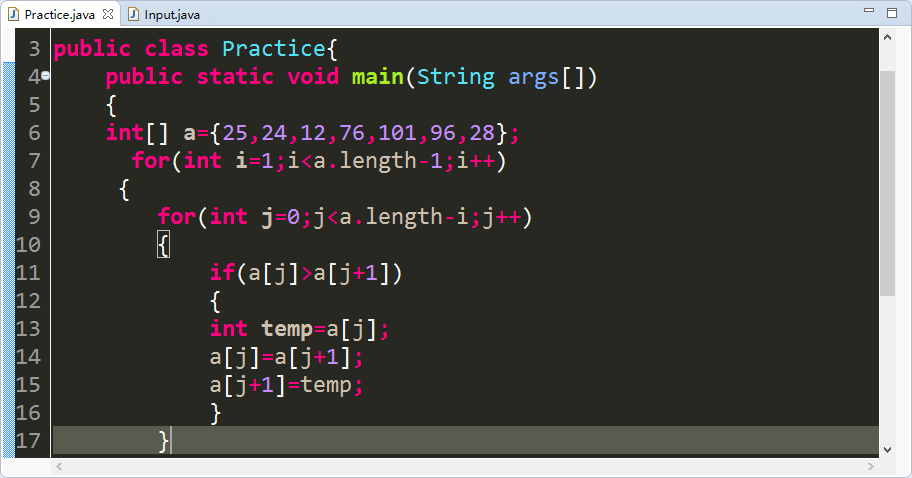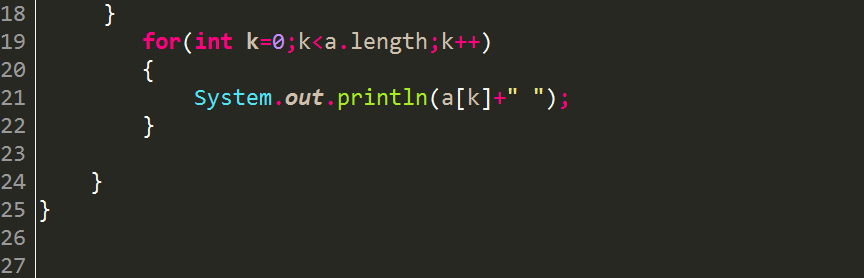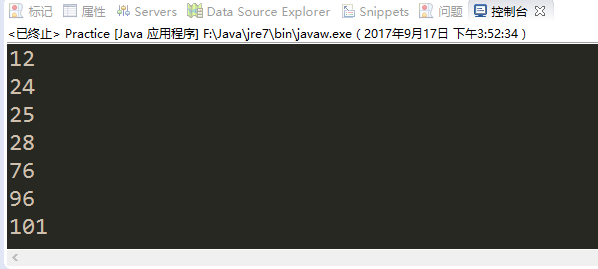# java等各种语言数组排序方法

java数组排序方法，常用的排序方法很多，有冒泡排序法、选择排序法、快速排序法，插入排序法等

public class Array {
public static void main(String args[]) {
int[] a = { 25, 24, 12, 76, 101, 96, 28 };
for (int i = 1; i < a.length - 1; i++)// 外层循环控制比较的轮数
{
for (int j = 0; j < a.length - i; j++)// 定义内层循环控制每一轮比较的次数
{
if (a[j] > a[j + 1])// 比较相邻元素
{
// 下面的三行代码用于交换两个元素
int temp = a[j];
a[j] = a[j + 1];
a[j + 1] = temp;
}
}
}
for (int k = 0; k < a.length; k++) {
System.out.println(a[k] + " ");
}
}
}©️2019 CSDN 皮肤主题: 技术黑板 设计师: CSDN官方博客